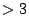# The Perfect-Graph Robust Algorithm Problem

An algorithm, which for any easily recognizable input, A, finds either an easily recognizable B or an easily recognizable C, is sometimes called a "robust algorithm" - to be distinguished from a non-robust algorithm, which, for any A without a B, finds a C. Either provides a proof of the "existentially polytime (EP) theorem": For any A, there exists a B or a C. In other words: For any A without a B, there is a C.

In [ MR 92i:68043], Jack Edmonds and Kathie Cameron advocated seeking a robust algorithm which, for any graph G, finds either a clique and colouring the same size or else finds an easily recognizable combinatorial obstruction to G being perfect. The obstruction might be specified to be a "alpha-omega partitioned subgraph", or it might be specified more particularly to be an odd hole or odd antihole.

Such an algorithm might be simpler than an algorithm for recognizing whether or not a graph is perfect, in view of precedents, and since what it would do is incomparable with perfect-graph recognition. Such an algorithm could end up giving a clique and colouring the same size in a non-perfect graph.

Here are two examples of similar problems which have been solved. Edmonds has given a simple robust algorithm which, for any graph G, either finds an odd cyclewith at most one chord (a defining obstruction to G being Meyniel) or else finds a clique and colouring the same size. This is an improvement on the non-robust algorithms of Hoang and Hertz which, assuming a graph is Meyniel, find a clique and colouring the same size. Edmonds' algorithm is much simpler than the Burlet-Fonlupt decomposition algorithm for recognizing Meyniel graphs, which was motivated by an interest in optimizing in Meyniel graphs, and which is used by Hoang and Hertz.

Conforti and Cornuejols give a complicated decomposition algorithm for recognizing whether or not a matrix is balanced. At about the same time, to motivate the advocacy of a robust algorithm for either node-colouring a graph or recognizing it to be not perfect, Cameron and Edmonds presented a simple algorithm which, for any 0-1 matrix M, either finds, where x is the largest number of ones in any row, an x-colouring of the columns so that the 1's of any row are in different coloured columns, or else finds "an odd hole" in M (the defining obstruction to M being balanced). This introduced the "EP - robust algorithm" paradigm which is followed in Edmonds' Meyniel-related algorithm, and is related to the Conforti-Cornuejols-Rao treatment of balanced matrices in the same way that Edmonds' is related to the Burlet-Fonlupt treatment of Meyniel graphs. Following the same paradigm we expect there to be a robust algorithm proving the SPCG, related in the same way to the Chudnovsky-Robertson-Seymour-Thomas decomposition of Berge graphs.

In conclusion, we know the following

EP Theorem 1: For any graph, there is either a clique and a colouring of the same size, or there is an alpha-omega partitioned subgraph (or both).

EP Theorem 2 (SPGT): For any graph, there is either a clique and a colouring of the same size, or there is a odd hole or odd antihole (or both). So: Give a combinatorial polytime algorithm to find what the EP theorem asserts to exist.

Contributed by Kathie Cameron and Jack Edmonds

Back to the main index for Perfect Graphs.